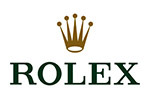### Rolex Clasp Codes

Here is information on how to ascertain your year which the Rolex clasp and bracelet was assembled.As example the following code stamped on the clasp above “CL1” which denotes as CL=2004 from the chart, with the number 1 as the month and “CL” is the year 2004. The bracelets will usually coincide with the watch year of manufacture. If there is “S”on the clasp that means clasp was changed in last service by Rolex AD.

A or VA = 1976

B or VB = 1977

C or VC = 1978

D or VD = 1979

E or VE = 1980

F or VF = 1981

G = 1982

H = 1983

I = 1984

J = 1985

K = 1986

L = 1987

M = 1988

N = 1989

O = 1990

P = 1991

Q = 1992

R = 1993

S = 1994

W or T = 1995

V = 1996

Z = 1997

U = 1998

X = 1999

AB = 2000

DE = 2001

DT = 2002

CL = 2004

MA = 2005

OP = 2006

EO = 2007

PJ = 2008

LT = 2009

RS = 2010

Random (3 Digit) = 2011